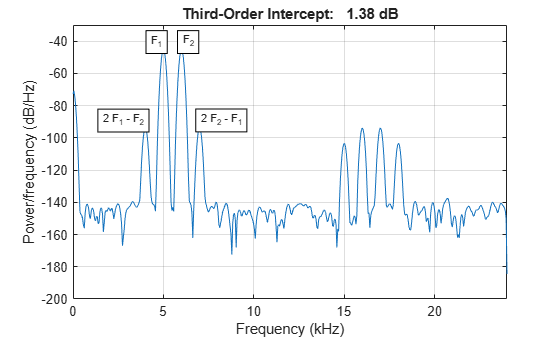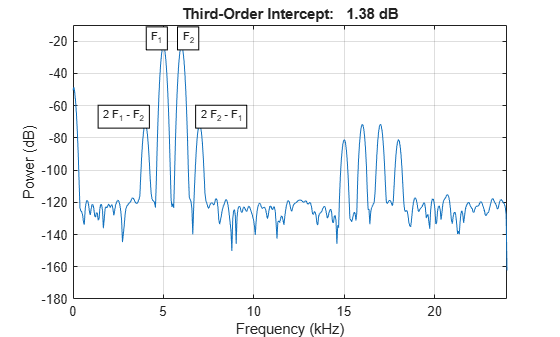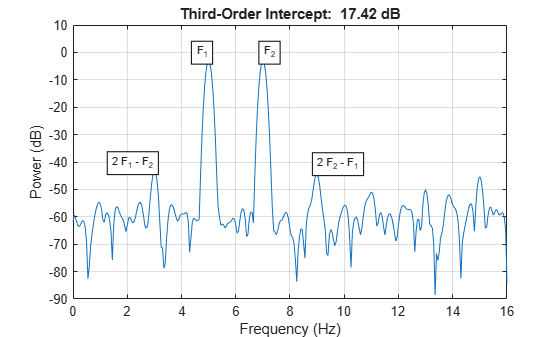# toi

Third-order intercept point

## Syntax

``oip3 = toi(x)``
``oip3 = toi(x,fs)``
``oip3 = toi(pxx,f,'psd')``
``oip3 = toi(sxx,f,rbw,'power')``
``````[oip3,fundpow,fundfreq,imodpow,imodfreq] = toi(___)``````
``toi(___)``

## Description

````oip3 = toi(x)` returns the output third-order intercept (TOI) point, in decibels (dB), of a real sinusoidal two-tone input signal, `x`. The computation is performed over a periodogram of the same length as the input using a Kaiser window with β = 38.```

example

````oip3 = toi(x,fs)` specifies the sample rate, `fs`. The default value of `fs` is `1`.```

example

````oip3 = toi(pxx,f,'psd')` specifies the input as a one-sided power spectral density (PSD), `pxx`, of a real signal. `f` is a vector of frequencies that corresponds to the vector of `pxx` estimates.```

example

````oip3 = toi(sxx,f,rbw,'power')` specifies the input as a one-sided power spectrum, `sxx`, of a real signal. `rbw` is the resolution bandwidth over which each power estimate is integrated.```

example

``````[oip3,fundpow,fundfreq,imodpow,imodfreq] = toi(___)``` also returns the power, `fundpow`, and frequencies, `fundfreq`, of the two fundamental sinusoids. It also returns the power, `imodpow`, and frequencies, `imodfreq`, of the lower and upper intermodulation products. This syntax can use any of the input arguments in the preceding syntaxes.```

example

````toi(___)` with no output arguments plots the spectrum of the signal and annotates the lower and upper fundamentals (f1, f2) and intermodulation products (2f1 – f2, 2f2 – f1). Higher harmonics and intermodulation products are not labeled. The TOI appears above the plot.```

## Examples

collapse all

Create a two-tone sinusoid with frequencies ${f}_{1}=5$ kHz and ${f}_{2}=6$ kHz, sampled at 48 kHz. Make the signal nonlinear by feeding it to a polynomial. Add noise. Set the random number generator to the default settings for reproducible results. Compute the third-order intercept point. Verify that the intermodulation products occur at $2{f}_{2}-{f}_{1}=4$ kHz and $2{f}_{1}-{f}_{2}=7$ kHz.

```rng default fi1 = 5e3; fi2 = 6e3; Fs = 48e3; N = 1000; x = sin(2*pi*fi1/Fs*(1:N))+sin(2*pi*fi2/Fs*(1:N)); y = polyval([0.5e-3 1e-7 0.1 3e-3],x)+1e-5*randn(1,N); [myTOI,Pfund,Ffund,Pim3,Fim3] = toi(y,Fs); myTOI,Fim3```
```myTOI = 1.3844 ```
```Fim3 = 1×2 103 × 4.0002 6.9998 ```

Create a two-tone sinusoid with frequencies 5 kHz and 6 kHz, sampled at 48 kHz. Make the signal nonlinear by evaluating a polynomial. Add noise. Set the random number generator to the default settings for reproducible results.

```rng default fi1 = 5e3; fi2 = 6e3; Fs = 48e3; N = 1000; x = sin(2*pi*fi1/Fs*(1:N))+sin(2*pi*fi2/Fs*(1:N)); y = polyval([0.5e-3 1e-7 0.1 3e-3],x)+1e-5*randn(1,N);```

Evaluate the periodogram of the signal using a Kaiser window. Compute the TOI using the power spectral density. Plot the result.

```w = kaiser(numel(y),38); [Sxx, F] = periodogram(y,w,N,Fs,'psd'); [myTOI,Pfund,Ffund,Pim3,Fim3] = toi(Sxx,F,'psd')```
```myTOI = 1.3843 ```
```Pfund = 1×2 -22.9133 -22.9132 ```
```Ffund = 1×2 103 × 5.0000 6.0000 ```
```Pim3 = 1×2 -71.4868 -71.5299 ```
```Fim3 = 1×2 103 × 4.0002 6.9998 ```
`toi(Sxx,F,'psd');`Create a two-tone sinusoid with frequencies 5 kHz and 6 kHz, sampled at 48 kHz. Make the signal nonlinear by evaluating a polynomial. Add noise. Set the random number generator to the default settings for reproducible results.

```rng default fi1 = 5e3; fi2 = 6e3; Fs = 48e3; N = 1000; x = sin(2*pi*fi1/Fs*(1:N))+sin(2*pi*fi2/Fs*(1:N)); y = polyval([0.5e-3 1e-7 0.1 3e-3],x)+1e-5*randn(1,N);```

Evaluate the periodogram of the signal using a Kaiser window. Compute the TOI using the power spectrum. Plot the result.

```w = kaiser(numel(y),38); [Sxx,F] = periodogram(y,w,N,Fs,'power'); toi(Sxx,F,enbw(w,Fs),'power')``````ans = 1.3844 ```

Generate 640 samples of a two-tone sinusoid with frequencies 5 Hz and 7 Hz, sampled at 32 Hz. Make the signal nonlinear by evaluating a polynomial. Add noise with standard deviation 0.01. Set the random number generator to the default settings for reproducible results. Compute the third-order intercept point. Verify that the intermodulation products occur at $2{f}_{2}-{f}_{1}=9\phantom{\rule{0.2777777777777778em}{0ex}}Hz$ and $2{f}_{1}-{f}_{2}=3\phantom{\rule{0.2777777777777778em}{0ex}}Hz$.

```rng default x = sin(2*pi*5/32*(1:640))+cos(2*pi*7/32*(1:640)); q = x + 0.01*x.^3 + 1e-2*randn(size(x)); [myTOI,Pfund,Ffund,Pim3,Fim3] = toi(q,32)```
```myTOI = 17.4230 ```
```Pfund = 1×2 -2.8350 -2.8201 ```
```Ffund = 1×2 5.0000 7.0001 ```
```Pim3 = 1×2 -43.1362 -43.5211 ```
```Fim3 = 1×2 3.0015 8.9744 ```

Generate 640 samples of a two-tone sinusoid with frequencies 5 Hz and 7 Hz, sampled at 32 Hz. Make the signal nonlinear by evaluating a polynomial. Add noise with standard deviation 0.01. Set the random number generator to the default settings. Plot the spectrum of the signal. Display the fundamentals and the intermodulation products. Verify that the latter occur at 9 Hz and 3 Hz.

```rng default x = sin(2*pi*5/32*(1:640))+cos(2*pi*7/32*(1:640)); q = x + 0.01*x.^3 + 1e-2*randn(size(x)); toi(q,32)``````ans = 17.4230 ```

## Input Arguments

collapse all

Real-valued sinusoidal two-tone signal, specified as a row or column vector.

Example: ```polyval([0.01 0 1 0],sum(sin(2*pi*[5 7]'*(1:640)/32))) + 0.01*randn([1 640])```

Data Types: `double` | `single`

Sample rate, specified as a positive real scalar. The sample rate is the number of samples per unit time. If the unit of time is seconds, then the sample rate has units of hertz.

Data Types: `double` | `single`

One-sided power spectral density estimate, specified as a real-valued, nonnegative row or column vector.

The power spectral density must be expressed in linear units, not decibels. Use `db2pow` to convert decibel values to power values.

Example: ```[pxx,f] = periodogram(cos(pi./[4;2]*(0:159))'+randn(160,2))``` specifies the periodogram PSD estimate of a noisy two-channel sinusoid sampled at 2π Hz and the frequencies at which it is computed.

Data Types: `double` | `single`

Cyclical frequencies corresponding to the one-sided PSD estimate, `pxx`, specified as a row or column vector. The first element of `f` must be 0.

Data Types: `double` | `single`

Power spectrum, specified as a real-valued nonnegative row or column vector.

The power spectrum must be expressed in linear units, not decibels. Use `db2pow` to convert decibel values to power values.

Example: ```[sxx,w] = periodogram(cos(pi./[4;2]*(0:159))'+randn(160,2),'power')``` specifies the periodogram power spectrum estimate of a two-channel sinusoid embedded in white Gaussian noise and the normalized frequencies at which it is computed.

Data Types: `double` | `single`

Resolution bandwidth, specified as a positive scalar. The resolution bandwidth is the product of the frequency resolution of the discrete Fourier transform and the equivalent noise bandwidth of the window.

Data Types: `double` | `single`

## Output Arguments

collapse all

Output third-order intercept point of a sinusoidal two-tone signal, returned as a real-valued scalar expressed in decibels. If the second primary tone is the second harmonic of the first primary tone, then the lower intermodulation product is at zero frequency. The function returns `NaN` in those cases.

Data Types: `double` | `single`

Power contained in the two fundamental sinusoids of the input signal, returned as a real-valued two-element row vector.

Data Types: `double` | `single`

Frequencies of the two fundamental sinusoids of the input signal, returned as a real-valued two-element row vector.

Data Types: `double` | `single`

Power contained in the lower and upper intermodulation products of the input signal, returned as a real-valued two-element row vector.

Data Types: `double` | `single`

Frequencies of the lower and upper intermodulation products of the input signal, returned as a real-valued two-element row vector.

Data Types: `double` | `single`

 Kundert, Kenneth S. “Accurate and Rapid Measurement of IP2 and IP3.” May, 2002. https://www.designers-guide.org/Analysis/intercept-point.pdf.